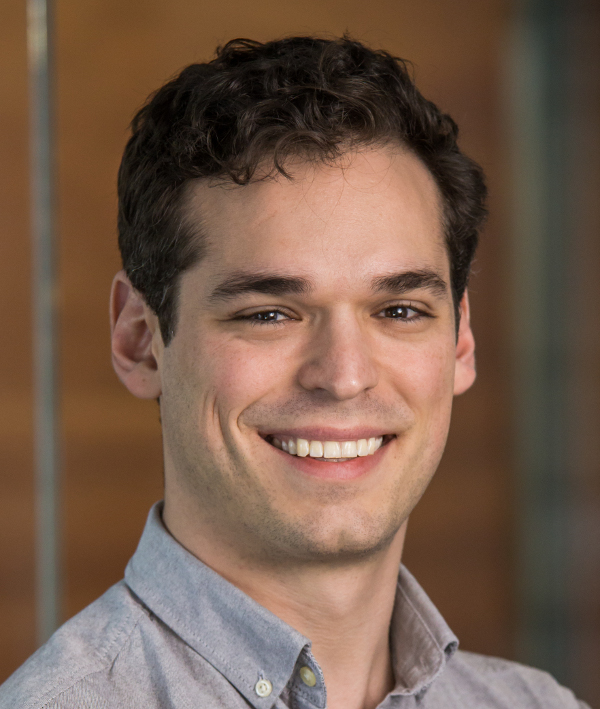Zak Kincaid I'm an associate professor at Princeton University. Email: zkincaid@cs.princeton.edu Office: Computer Science Building, Room 219 Address: 35 Olden Street, Princeton, NJ 08540

# news

• "When Less is More: Consequence-finding in a Weak Theory of Arithmetic" with Nicolas Koh and Shaowei Zhu to appear at POPL 2023
• "Reflections on Termination of Linear Loops" with Shaowei Zhu to appear at CAV 2021
• Tom Reps and I will deliver a tutorial on algebraic program analysis at CAV 2021
• "Termination Analysis without the Tears" with Shaowei Zhu to appear at PLDI 2021

# research

My main research interests are in program analysis, logic, and programming languages. I have active research projects that aim to make program analysis compositional and robust.

# selected publications (full list at dblp)

 When Less is More: Consequence-finding in a Weak Theory of Arithmetic with Nicolas Koh and Shaowei Zhu. POPL 2023. This paper presents a theory of non-linear integer/real arithmetic and algorithms for reasoning about this theory. The theory can be conceived of as an extension of linear integer/real arithmetic with a weakly-axiomatized multiplication symbol, which retains many of the desirable algorithmic properties of linear arithmetic. In particular, we show that the conjunctive fragment of the theory can be eﬀectively manipulated (analogously to the usual operations on convex polyhedra, the conjunctive fragment of linear arithmetic). As a result, we can solve the following consequence-ﬁnding problem: given a ground formula F, ﬁnd the strongest conjunctive formula that is entailed by F. As an application of consequence-ﬁnding, we give a loop invariant generation algorithm that is monotone with respect to the theory and (in a sense) complete.PDF

 Reflections on Termination of Linear Loops with Shaowei Zhu. CAV 2021. This paper shows how techniques for linear dynamical systems can be used to reason about the behavior of general loops. We present two main results. First, we show that every loop that can be expressed as a transition formula in linear integer arithmetic has a best model as a deterministic affine transition system. Second, we show that for any linear dynamical system f with integer eigenvalues and any integer arithmetic formula G, there is a linear integer arithmetic formula that holds exactly for the states of F for which G is eventually invariant. Combining the two, we develop a monotone conditional termination analysis for general loops.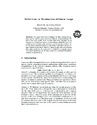PDF

 Termination Analysis Without the Tears with Shaowei Zhu. PLDI 2021. Termination is undecidable, and so algorithms for termination analysis typically either (1) provide strong behavior guarantees, but work in limited circumstances or (2) are broadly applicable, but have weak behavior guarantees. This paper presents ComPACT, a practical termination analysis that is both compositional (the result of analyzing a composite program is a function of the analysis results of its components) and monotone (“more information into the analysis yields more information out”). The key contributions are (1) an extension of Tarjan's method for solving path problems in graphs to solve infinite path problems and (2) a collection of monotone conditional termination analyses based on this framework. We demonstrate that our tool ComPACT is competitive with state-of-the-art termination tools while providing stronger behavioral guarantees.PDF

 Templates and Recurrences: Better Together with Jason Breck, John Cyphert, and Tom Reps. PLDI 2020. Two prominent approaches to generating numerical program invariants are templated-based methods, which reduce invariant generation to constraint solving by fixing a template invariant and solving for the unknowns, and recurrence-based methods, which compute invariants by extracting recurrence relations from code and computing their closed forms. In this paper, we combine these two approaches and obtain a technique that overcomes some of the limitations of each. It uses templates in which the unknowns are functions rather than numbers, and the constraints on the unknowns are recurrences. The technique synthesizes invariants involving polynomials, exponentials, and logarithms, even in the presence of complex control-flow, including any combination of loops, branches, and (possibly non-linear) recursion.PDF

 Loop Summarization with Rational Vector Addition Systems with Jake Silverman. CAV 2019. This paper presents a technique for computing numerical loop summaries. The method synthesizes a rational vector addition system with resets (Q-VASR) that simulates the action of an input loop, and then uses the reachability relation of that Q-VASR to over-approximate the behavior of the loop. The key technical problem solved in this paper is to automatically synthesize a Q-VASR that is a best abstraction of a given loop in the sense that (1) it simulates the loop and (2) it is simulated by any other Q-VASR that simulates the loop. Since our loop summarization scheme is based on computing the exact reachability relation of a best abstraction of a loop, we can make theoretical guarantees about its behavior. Moreover, we show experimentally that the technique is precise and performant in practice.PDF

 A Practical Algorithm for Structure Embedding with Charlie Murphy. VMCAI 2019. This paper presents an algorithm for the structure embedding problem: given two finite first-order structures over a common relational vocabulary, does there exist an injective homomorphism from one to the other? The structure embedding problem is NP-complete in the general case, but for monadic structures (each predicate has arity at most 1) we observe that it can be solved in polytime by reduction to bipartite graph matching. Our algorithm, MatchEmbeds, extends the bipartite matching approach to the general case by using it as the foundation of a backtracking search procedure. We show that MatchEmbeds outper- forms state-of-the-art SAT, CSP, and subgraph isomorphism solvers on difficult random instances and significantly improves the performance of a client model checker for multi-threaded programs.PDF

 Closed Forms for Numerical Loops with Jason Breck, John Cyphert, and Tom Reps. POPL 2019. This paper investigates the problem of reasoning about non-linear behavior of simple numerical loops. Our approach builds on classical techniques for analyzing the behavior of linear dynamical systems. It is well-known that a closed-form representation of the behavior of a linear dynamical system can always be expressed using algebraic numbers, but this approach can create formulas that present an obstacle for automated-reasoning tools. This paper characterizes when linear loops have closed forms in simpler theories that are more amenable to automated reasoning. The algorithms for computing closed forms described in the paper avoid the use of algebraic numbers, and produce closed forms expressed using polynomials and exponentials over rational numbers. We show that the logic for expressing closed forms is decidable, yielding decision procedures for verifying safety and termination of a class of numerical loops over rational numbers. We also show that the procedure for computing closed forms for this class of numerical loops can be usedxc to over-approximate the behavior of arbitrary numerical programs (with unrestricted control flow, non-deterministic assignments, and recursive procedures).PDF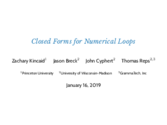Slides

 Refinement of Path Expressions for Static Analysis with John Cyphert, Jason Breck, and Tom Reps. POPL 2019. Algebraic program analyses compute information about a program's behavior by first (a) computing a valid path expression and then (b) interpreting the path expression in a semantic algebra that defines the analysis. There are an infinite number of different regular expressions that qualify as valid path expressions, which raises the question: which one should we choose? While any choice yields a sound result, for many analyses the choice can have a drastic effect on the precision of the results obtained. In this paper, we develop an algorithm that takes as input a valid path expression E, and returns a valid path expression E' that is guaranteed to yield analysis results that are at least as good as those obtained using E.PDF

 Numerical Invariants via Abstract Machines. Invited talk at SAS 2018. This talk presents an overview of a line of recent work on generating non-linear numerical invariants for loops and recursive procedures. The method is compositional in the sense that it operates by breaking the program into parts, analyzing each part independently, and then combining the results. The fundamental challenge is to devise an effective method for analyzing the behavior of a loop given the results of analyzing its body. The key idea is to separate the problem into two: first we approximate the loop dynamics by an abstract machine, and then symbolically compute the reachability relation of the abstract machine.PDF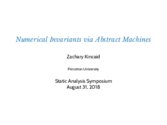Slides

 Non-Linear Reasoning For Invariant Synthesis with Jason Breck, John Cyphert, and Tom Reps. POPL 2018. An appealing approach to non-linear invariant generation is to exploit the powerful recurrence-solving techniques that have been developed in the field of computer algebra. However, there is a gap between the capabilities of recurrence solvers and the needs of program analysis: (1) loop bodies are not merely systems of recurrence relations---they may contain conditional branches, nested loops, non-deterministic assignments, etc., and (2) a client program analyzer must be able to reason about the closed-form solutions produced by a recurrence solver (e.g., to prove assertions). This paper presents a method for generating non-linear invariants for general loops by analyzing recurrence relations. The key components are an abstract domain for reasoning about non-linear arithmetic, a semantics-based method for extracting recurrence relations from loop bodies, and a recurrence solver that avoids closed forms that involve complex or irrational numbers.PDFSlides

 Strategy Synthesis for Linear Arithmetic Games with Azadeh Farzan. POPL 2018. This paper studies the strategy synthesis problem for games defined within the theory of linear rational arithmetic. Two types of games are considered. A satisfiability game, described by a quantified formula, is played by two players that take turns instantiating quantifiers. The objective of each player is to prove (or disprove) satisfiability of the formula. A reachability game, described by a pair of formulas defining the legal moves of each player, is played by two players that take turns choosing positions---rational vectors of some fixed dimension. The objective of each player is to reach a position where the opposing player has no legal moves (or to play the game forever). We give a complete algorithm for synthesizing winning strategies for satisfiability games and a semi-algorithm for synthesizing winning strategies for reachability games.PDF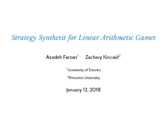Slides

 A Symbolic Decision Procedure for Symbolic Alternating Automata with Loris D'Antoni, and Fang Wang. MFPS 2017. We introduce Symbolic Alternating Finite Automata (s-AFA) as a succinct and decidable model for describing sets of finite sequences over arbitrary alphabets. Boolean operations over s-AFAs have linear complexity, which contrasts the quadratic cost of intersection and union for non-alternating symbolic automata. Due to this succinctness, emptiness and equivalence checking are PSpace-hard. We introduce an algorithm for checking the equivalence of two s-AFAs based on bisimulation up to congruence. This algorithm exploits the power of SAT solvers to efficiently search the state space of the s-AFAs.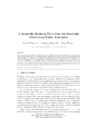PDF

 Compositional Recurrence Analysis Revisited with Jason Breck, Ashkan Boroujeni, and Tom Reps. PLDI 2017. Compositional recurrence analysis (CRA) is a static-analysis method based on a combination of symbolic analysis and abstract interpretation. CRA computes the meaning of a procedure following Tarjan’s path-expression method: first compute a regular expression recognizing a set of paths through the procedure, then interpret that regular expression within a suitable semantic algebra. This paper introduces ICRA, an extension of CRA to recursive procedures. ICRA overcomes the “impedance mismatch” between CRA, which relies on representing program paths with regular languages, and the context-free-language underpinnings of context-sensitive analysis.PDFSlides

 Linear Arithmetic Satisfiability via Strategy Improvement with Azadeh Farzan. IJCAI 2016. This article presents a decision procedure for the theory of linear rational arithmetic (and linear integer arithmetic), including quantifiers. The algorithm is based on synthesizing winning strategies for quantified formulas (interpreted as satisfiability games) by mutual strategy improvement.PDF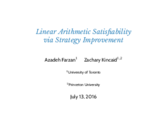Slides

 Proving Liveness of Parameterized Programs with Azadeh Farzan and Andreas Podelski. LICS 2016. Correctness of multi-threaded programs typically requires that they satisfy liveness properties. For example, a program may require that no thread is starved of a shared resource, or that all threads eventually agree on a single value. This paper presents a method for proving that such liveness properties hold. Two particular challenges addressed in this work are that (1) the correctness argument may rely on global behaviour of the system (e.g., the correctness argument may require that all threads collectively progress towards "the good thing" rather than one thread progressing while the others do not interfere), and (2) such programs are often designed to be executed by any number of threads, and the desired liveness properties must hold regardless of the number of threads that are active in the program.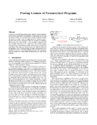PDF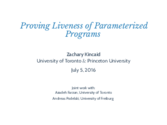Slides

 Compositional Recurrence Analysis with Azadeh Farzan. FMCAD 2015. This paper presents a new method for automatically generating numerical invariants for imperative programs. The procedure computes a transition formula which over-approximates the behaviour of a given input program. It is compositional in the sense that it operates by decomposing the program into parts, computing a transition formula for each part, and then composing them. Transition formulas for loops are computed by extracting recurrence relations from a transition formula for the loop body and then computing their closed forms. Experimentation demonstrates that this method is competitive with leading verification techniques.PDF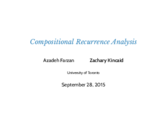Slides

 Spatial Interpolants with Aws Albarghouthi, Josh Berdine, and Byron Cook. ESOP 2015. We propose SplInter, a new technique for proving properties of heap-manipulating programs that marries (1) a new separation logic-based analysis for heap reasoning with (2) an interpolation-based technique for refining heap-shape invariants with data invariants. SplInter is property directed, precise, and produces counterexample traces when a property does not hold. Using the novel notion of spatial interpolants modulo theories, SplInter can infer complex invariants over general recursive predicates, e.g., of the form "all elements in a linked list are even" or "a binary tree is sorted."PDF

 Proof Spaces for Unbounded Parallelism with Azadeh Farzan and Andreas Podelski. POPL 2015. In this paper, we describe proof spaces, a proof system for verifying safety properties for multi-threaded programs in which the number of executing threads is not statically bounded. Our development of this proof system is motivated by the question of how to generalize a proof of correctness (perhaps obtained from a verifier for sequential programs) of a some finite set of example traces so that the correctness argument applies to all traces of the program. We show that proof spaces are complete relative to the inductive assertion method, and give algorithms for automating them.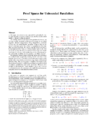PDF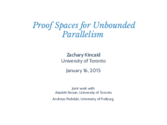Slides

 Consistency Analysis of Decision-Making Programs with Swarat Chaudhuri and Azadeh Farzan. POPL 2014. Applications in many areas of computing make discrete decisions under uncertainty; for example, the application may rely on limited numerical precision in input, or on input or sensory data. While an application executing under uncertainty cannot be relied upon to make decisions which are correct with respect to a given world, it is desirable that their decisions are at least consistent (i.e., correct with respect to some possible world). This paper presents a sound, automatic program analysis for verifying program consistency.PDF

 Proofs that count with Azadeh Farzan and Andreas Podelski. POPL 2014. Counting arguments are among the most basic methods of proof in mathematics. Within the field of formal verification, they are useful for reasoning about programs with infinite control, such as programs with an unbounded number of threads, or (concurrent) programs with recursive procedures. While counting arguments are common in informal, hand-written proofs of such programs, there are no fully automated techniques to construct counting arguments. The key questions involved in automating counting arguments are: how to decide what should be counted?, and how to decide when a counting argument is valid? In this paper, we present a technique for automatically constructing and checking counting arguments, which includes novel solutions to these questions.PDF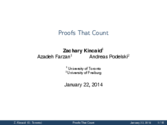Slides

 Symbolic Optimization with SMT solvers with Yi Li, Aws Albarghouthi, Arie Gurfinkel and Marsha Chechik. POPL 2014. The rise in efficiency of Satisfiability Modulo Theories (SMT) solvers has created numerous uses for them in programming languages: software verification, program synthesis, functional programming, refinement types, etc. SMT solvers are effective at finding arbitrary satisfying assignments for formulae, but for some applications it is necessary to find an assignment that optimizes (minimizes/maximizes) certain criteria. We present an efficient SMT-based optimization algorithm for objective functions in the theory of linear real arithmetic.PDF

 Duet: static analysis for unbounded parallelism with Azadeh Farzan. CAV 2013. Duet is a static analysis tool for concurrent programs in which the number of executing threads is not statically bounded. It has a modular architecture, which is based on separating the invariant synthesis problem in two subtasks: (1) data dependence analysis, which is used to construct a data flow model of the program, and (2) interpretation of the data flow model over a (possibly infinite) abstract domain, which generates invariants. This separation of concerns allows researchers working on data dependence analysis and abstract domains to combine their efforts toward solving the challenging problem of static analysis for unbounded concurrency.PDF

 Recursive program synthesis with Aws Albargouthi and Sumit Gulwani. CAV 2013. Program synthesis from input-output examples has the power of extending the range of computational tasks achievable by end-users who have no programming knowledge, but can articulate their desired computations by describing input-output behaviour. In this paper we present Escher, an algorithm that interacts with the user via input-output examples to synthesize recursive programs. Escher is parameterized by the components that can be used in the program, thus providing a generic synthesis algorithm that can be instantiated to suit different domains. Escher adopts a novel search strategy through the space of programs that utilizes special datastructures for inferring conditionals and synthesizing recursive procedures.PDF

 Inductive data flow graphs with Azadeh Farzan and Andreas Podelski. POPL 2013. We propose inductive data flow graphs, data flow graphs with incorporated inductive assertions, as the basis of an approach to verifying concurrent programs. An inductive data flow graph accounts for a set of dependencies between program events, and therefore stands as a representation for the set of executions which give rise to these dependencies. By representing information about dependencies rather than control flow, inductive data flow graphs can yield very succinct proofs. Our strategy for verifying concurrent programs defers reasoning about control to the proof checking step, a purely combinatorial problem, thus avoiding the need to reason about data and control simultaneously.PDFSlides

 Verification of parameterized concurrent programs by modular reasoning about data and control with Azadeh Farzan. POPL 2012. We consider the problem of verifying thread-state properties of multithreaded programs in which the number of active threads cannot be statically bounded. Our approach is based on decomposing the task into two modules, where one reasons about data and the other reasons about control. The two modules are incorporated into a feedback loop, so that the abstractions of data and control are iteratively coarsened as the algorithm progresses (that is, they become weaker) until a fixed point is reached. This version fixes some typographical errors that appeared in the printed version - thanks to Chinmay Narayan, Suvam Mukherjee, and Deepak D'Souza for finding them.PDF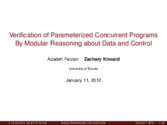Slides

 Compositional bitvector analysis for concurrent programs with nested locks with Azadeh Farzan. SAS 2010. We propose a new technique for bitvector data flow analysis for concurrent programs. Our algorithm works for concurrent programs that synchronize via nested locks. We give a compositional algorithm that first computes thread summaries and then efficiently combines them to solve the dataflow analysis problem. We show that this algorithm computes precise solutions (meet over all paths) to bitvector problems.PDF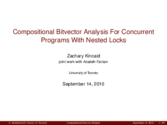Slides

# teaching

Fall 2021 - COS 516 / ELE 516: Automated Reasoning about Software
Past:

# activities

• PLDI 2022: Program Committee.
• POPL 2021: Program Committee.
• CAV 2020: Program Committee.
• PLDI 2020: External Review Committee.
• POPL 2019-2020: Workshop co-chair.
• OBT 2018: Program Committee.
• CAV 2018: Program Committee.
• VSTTE 2017: Program Committee.
• CAV 2017: Program Committee.
• PLDI 2017: Program Committee.
• POPL 2017: Program Committee.
• CAV 2016: Workshop chair/Program Committee.
• PLDI 2016: External Review Committee.
• TACAS 2016: Program Committee.
• SSS 2015: Program Committee
• Tiny Transactions on Computer Science vol. 3.: Program Committee
• PLDI 2014: Artifact Evaluation Committee